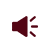• ISSN 1001-1455  CN 51-1148/O3
• EI、Scopus、CA、JST收录
• 力学类中文核心期刊
• 中国科技核心期刊、CSCD统计源期刊引用本文: 郭宏展, 张衍, 王筱蓉. 氢气-甲烷-乙醇混合燃料的爆炸压力特性[J]. 爆炸与冲击.GUO Hongzhan, ZHANG Yan, WANG Xiaorong. Explosion pressure characteristics of hydrogen-methane-ethanol mixtures[J]. Explosion And Shock Waves. doi: 10.11883/bzycj-2023-0224
 Citation: GUO Hongzhan, ZHANG Yan, WANG Xiaorong. Explosion pressure characteristics of hydrogen-methane-ethanol mixtures[J]. Explosion And Shock Waves.## 氢气-甲烷-乙醇混合燃料的爆炸压力特性

##### doi: 10.11883/bzycj-2023-0224

###### 通讯作者: 王筱蓉（1982－　 ），女，博士，教授，ghz18755780815@163.com
• 中图分类号: O389; O642.1

## Explosion pressure characteristics of hydrogen-methane-ethanol mixtures

• 摘要: 在初始温度为400 K，不同的初始压力（0.1、0.2、0.4 MPa）、乙醇体积分数（20%，50%，80%）和当量比（0.8～1.4）下，采用定容燃烧弹对氢气-甲烷-乙醇的爆炸特性进行研究。将实验中获得的原始电压信号转换为压力数据并进行高斯滤波降噪处理，通过爆炸压力、压力上升率、爆炸指数和爆炸时间等相关参数来分析爆炸特性，这些参数可以用于评估可燃物爆炸强烈程度，同时对燃料的层流燃烧速度、敏感性和反应路径进行分析。结果表明：随着初始压力的增加，预混燃料的爆炸压力峰值、最大压力上升速率、爆炸指数和爆炸时间均显著增加。乙醇比例的增加会降低最大压力上升速率和爆炸指数，但会增加爆炸压力和爆炸时间。燃料在不同的初始压力和乙醇比例下，总在当量比为1.2～1.3之间达到爆炸压力峰值、最大压力上升速率、爆炸指数的极大值和爆炸时间的极小值。此外，灵敏度分析表明预混燃料的爆炸反应程度与燃烧过程中生成的H和OH自由基数量有很大关系。总体而言，研究获得的爆炸指数在大多数工况下均低于20 MPa·m/s，表明该燃料处于相对安全水平。
• 图  1  实验系统结构

Figure  1.  Structure schematic of experimental system

图  2  爆炸参数示意图

Figure  2.  Schematic diagram of explosion parameters

图  3  爆炸压力随时间变化规律

Figure  3.  Explosion pressure changing with time

图  4  p0=0.4 MPa、$\varPhi$=1时C2H5OH体积分数φ对爆炸压力曲线的影响

Figure  4.  Effect of the volume fraction of C2H5OH (φ) on explosion pressure while p0=0.4 MPa and $\varPhi$=1

图  5  φ=20%、$\varPhi$=1时初始压力p0对爆炸压力曲线的影响

Figure  5.  Effect of the initial pressure (p0) on explosion pressure while φ=20% and $\varPhi$=1

图  6  当量比$\varPhi$对压力峰值pmax的影响

Figure  6.  Effect of the equivalence ratio $\varPhi$on the peak pressure pmax

图  7  初始压力p0对压力峰值pmax的影响

Figure  7.  Effect of initial pressure p0 on the peak pressure pmax

图  8  爆炸压力上升率随时间的变化曲线

Figure  8.  Pressure rising rate by explosion changing with time

图  9  不同C2H5OH体积分数φ下压力上升速率曲线

Figure  9.  Pressure rise rate changing with time at different ethnol volume fractions (φ)

图  10  不同初始压力p0条件下的爆炸压力上升率曲线

Figure  10.  Explosion pressure rise rate curve at different initial pressures (p0)

图  11  火焰不稳定图像

Figure  11.  Flame instability image

图  12  最大压力上升速率(dp/dt)max和爆炸指数KG

Figure  12.  Maximum pressure rise rate ((dp/dt)max) and explosion index (KG)

图  13  初始压力p0对爆炸压力上升率峰值（(dp/dt)max）的影响

Figure  13.  Effect of initial pressure (p0) on the peak rate of explosion pressure rise ((dp/dt)max)

图  14  爆炸时间随当量比的变化曲线

Figure  14.  Curve of explosion time with equivalence ratio

图  15  初始压力对爆炸时间的影响

Figure  15.  The effect of initial pressure on explosion time

图  16  本文方法得到的层流燃烧速度与文献[38, 40-41]的对比

Figure  16.  Laminar burning velocity according to this paper compared with the result from reference [38, 40-41]

图  17  H2/CH4/C2H5OH实验和模拟层流燃烧速度的比较

Figure  17.  Comparison of experimental and simulated results of laminar combustion velocity

图  18  不同C2H5OH体积分数φ下的灵敏度数据（400 K, 0.1 MPa, Φ=1.2）

Figure  18.  Sensitivity coeffient at different vulume fractions (φ) under the condition of 400 K, 0.1 MPa, Φ=1.2)

图  19  不同初始压力p0下的灵敏度数据（400 K, φ=50%, Φ=1.2）

Figure  19.  Sensitivity coeffient of at different pressures under the condition of (400 K, φ=50%, Φ=1.2)

图  20  不同C2H5OH体积分数（φ）的反应路径图（0.1 MPa, 400 K, Φ=1.2）

Figure  20.  The reaction pathway due to different volume fractions (φ) under the condition of 0.1 MPa, 400 K and Φ=1.2

•点击查看大图
##### 计量
• 文章访问数:  43
• HTML全文浏览量:  12
• PDF下载量:  6
• 被引次数: 0
##### 出版历程
• 收稿日期:  2023-06-28
• 修回日期:  2023-08-30
• 网络出版日期:  2023-10-25

### 目录/下载:  全尺寸图片 幻灯片
• 分享
• 用微信扫码二维码

分享至好友和朋友圈